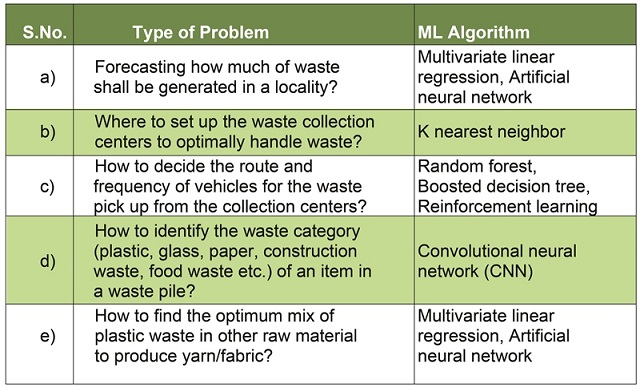# Machine Learning Applications in Waste Management –Part 1

Machine learning (ML) is being used in many industries to efficiently use data and train models to smartly execute the complex tasks. ML applications could also be used in many areas of waste management. Let’s us list down some of the challenging tasks of waste management which are data centric;

Forecasting how much of waste shall be generated in a locality? This can further be extended to how much of each type of waste shall be generated in a locality at any time of year?

-Where to set up the waste collection centers to optimally handle waste?

-How to decide the route and frequency of vehicles for the waste pick up from the collection centers?

-How to identify the waste category (plastic, glass, paper, construction waste, food waste etc.) of an item in a waste pile?

-How to find the optimum mix of plastic waste in other raw material to produce yarn/fabric?

Can you think of more problems of waste management which require large amount of data to be solved? Please mention those problems/tasks in the comment section of this article.

How machine learning can help us solve these problems? What are the different machine learning algorithms to solve these problems?

We can list some of the machine learning (ML) algorithms, suitable to solve each of the above problems, as below;### What is Machine Learning (ML)?

We can define it by listing its key features as below;

-It uses mathematical models to make inference

-Builds the mathematical models using given data/past experience

Tom Mitchell, famed Professor at Carnegie Mellon University defines Machine Learning as follows:

“A computer program is said to learn from experience E with respect to some class of tasks T and performance measure P, if its performance at tasks in T, as measured by P, improves with experience E. “

In fig.1 below, we can see that in machine learning, the model learns the formula/equation from the data (set of input and corresponding outputs) as opposed to usual computations, where we know the formula/equation and get the output by supplying the input to the formula.

### Types of Machine Learning Algorithms:

There are many algorithms of machine learning. It’s an ever-evolving field with new developments happening at leading research universities across the world and also in leading companies like Google’s DeepMind, Google Brain, Open AI Foundation, Tesla and Facebook etc. Primarily, the machine learning algorithm could be clubbed under the following three broad categories.

-Supervised Learning

-Unsupervised Learning

-Reinforcement Learning

When we have the labeled data (value of dependent variable ‘Yi’ is given for independent variables ‘Xi’ for each ith pair of data), then supervised learning techniques are used. Otherwise, unsupervised learning techniques are used. Reinforcement learning is a different type of machine learning where labeled/unlabeled data is not of primary importance and the learning happens through reward (+ve or -ve) based on the actions chosen by the agent in the machine learning model.

The machine learning algorithms mentioned in table 1 above could all be classified under supervised machine learning algorithms except the ‘K nearest neighbor’, which is an unsupervised machine learning algorithm.

In the next section, we shall discuss about each of the above types of machine learning techniques i.e.; supervised, unsupervised and reinforcement learning. We shall also discuss how can the waste management problems, mentioned in table 1 above, could be modelled and solved by the ML algorithms.